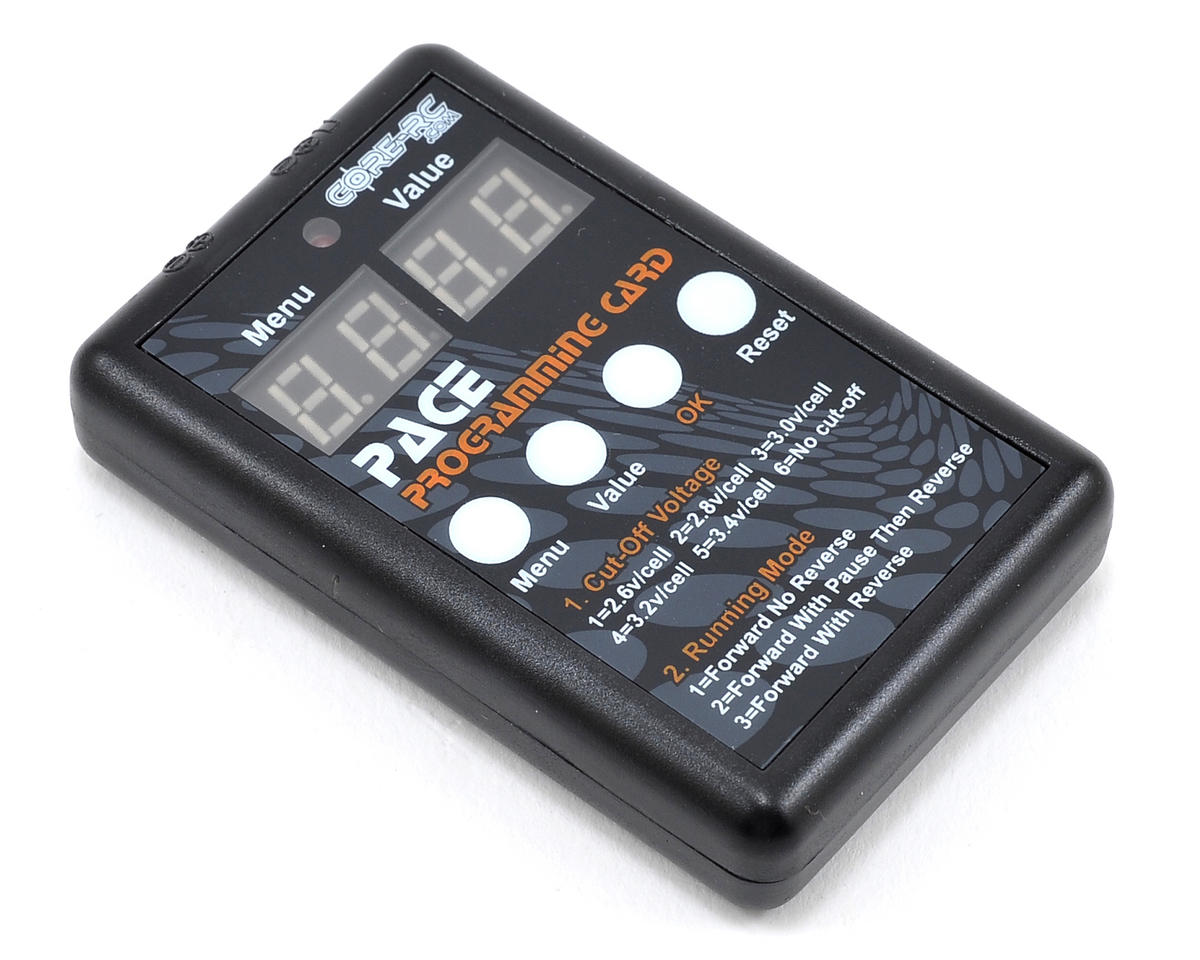For visually impaired users: Browse the streamlined version of our website.

# Core-RC PACE 45R Programming Card

Please be aware that pricing and product availability in-store may vary from what’s available online because each store is independently owned and operated.
Low Price: \$12.25
\$1.99 Flat Rate Shipping!This is the optional Core-RC Programming Card, and is intended for use with the Core-RC PACE 45R ESC.

Allows you to adjust the following settings:

• Cut-Off Voltage: 1=2.6v/cell  2=2.8v/cell  3=3.0v/cell 4=3.2v/cell  5=3.4v/cell  6=No cut-off
• Running Mode: 1=Forward No Reverse 2=Forward With Pause Then Reverse 3=Forward With Reverse
• Motor Timing: 1=Zero ‘Blinky’  2=Low  3=Normal 4=High   5=Very High
• Initial Acceleration: 1=Low  2=Medium  3=High 4=Very High
• Throttle Percent Reverse: 1=20%  2=30%  3=40% 4=50% 5=60% 6=70%  7=80%  8=90% 9=100%
• Throttle Limit: 1=0%  2=20%  3=30% 4=40% 5=50% 6=60%  7=70%  8=80% 9=90%
• Percentage Braking: 1=10%  2=20%  3=30% 4=40% 5=50% 6=60%  7=70%  8=80% 9=100%
• Percentage Drag Brake: 1=0%  2=4%  3=8% 4=12% 5=15% 6=20%  7=25%  8=30%
• Motor Rotation: 1=Normal  2=Reverse
• Neutral Range: 1=2%  2=3%  3=4% 4=5% 5=6%  6=10%

This product was added to our catalog on August 20, 2012